# ADC power-supply rejection, calculating allowed supply ripple

Other Parts Discussed in Post: ADC3444

In my previous blog, “Measuring PSRR in an ADC,” I looked at the methodology and techniques needed to measure the power-supply rejection ratio (PSRR). In this blog post, we’ll finish the analog-to-digital converter (ADC) characterization, interpret the results, and look in-depth at component selections to understand power-supply specification trade-offs versus ADC performance.

Let’s first start by looking at the ADC PSRR characterization. For continuity, we’ll use the ADC3444 as the example. The ADC3444 is a quad, 14-bit, 125MSPS pipeline ADC. Using the methodology developed in “Measuring PSRR in an ADC,” we created these PSRR plots:

• Analog VDD (AVDD) PSRR at DC and around fundamental versus frequency.
• AVDD PSRR versus fundamental input power.
• Digital VDD (DVDD) PSRR versus frequency.

As a reminder from the previous discussion, the AC signal of frequency f0 inserted in the ADC analog power supply (AVDD) is generating three spurs: one at DC at f0 and two around the single tone, at frequencies f ± f0. The same AC signal on the digital power supply of the ADC (DVDD) is at f0. See Figure 1.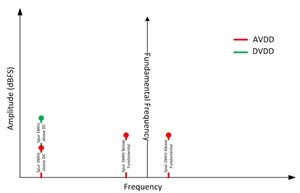Figure 1: Spurs location due to an AC signal present on the ADC power supply

The ADC3444 AVDD and DVDD supplies full PSRR characterization, as shown below. Note that the local bypass to each ADC was in place, with 0.1µF on each AVDD supply pin and 0.22µF on each DVDD supply pin, for a total of 1.3µF on AVDD and 0.88µF on DVDD. Figure 2 is the test configuration block diagram.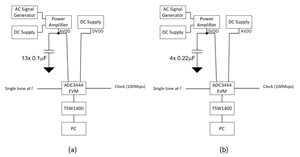Figure 2: (a) AVDD test circuit configuration; (b) DVDD test circuit configuration

Figure 3 shows the PSRR versus frequency for a fundamental at -2dBFS. Two conclusions jump out at you:

• The PSRR for the two spurs around the fundamental is 20dB worse than the PSRR at DC.
• Both PSRRs are flat to ~200kHz and are actually improving.

The worsening PSRR around the fundamental could suggest a dependency to the fundamental amplitude. So I measured the PSRR versus amplitude fundamental with a 500kHz ADC-supply AC signal (disturber signal).

The PSRR improvement versus frequency is not caused by the ADC PSRR, but by the disturber signal being attenuated by the bypass capacitors.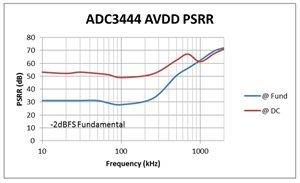Figure 3: AVDD PSRR vs. frequency

To verify that the PSRR of the AVDD supply is dependent on the fundamental, Figure 4 was measured. It shows a dB/dB dependency of the spur with the fundamental. In other terms, the disturber is present around the fundamental with a set dBc (dB below carrier) response. At DC, the disturber stays constant for any signal in the dynamic range of the ADC.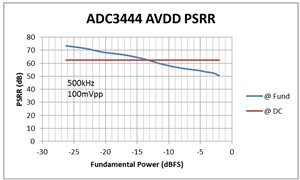I took the same approach for the digital power supply of the ADC, reflected in Figures 5 and 6. As expected, the digital-supply PSRR is much better than that of the analog supply by one order of magnitude, or 20dB. The presence of the bypass capacitor is also felt, but beyond 300kHz but does not last as long as on the analog supply. There is also no dependency on the amplitude of the fundamental.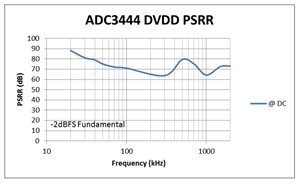Figure 5: DVDD PSRR versus frequency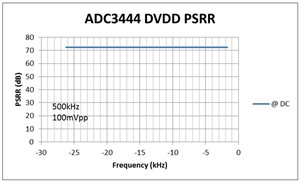Figure 6: DVDD PSRR versus input power

This was an interesting exercise, but what conclusion can we draw from the results?

The first conclusion is that the architecture used in the ADC3444 is as mostly sensitive on the analog power supply. Keep in mind that the above results are typical and a guardband should be added. Since 28dB is the worst result at -2dBFS and the PSRR is degraded dB/dB, the full-swing 0dBFS would have 26dB PSRR. Consider a guardband of at least 10dB for overtemperature and overprocess variations, leaving the minimum PSRR for the ADC3444 AVDD to be 16dB. The 10dB guardband is an estimate and requires additional characterization to ensure an adequate performance level.

Using the same equations as those found in “Measuring PSRR in an ADC,” see Equations 1 and 2 below. It is now possible to estimate the maximum allowable ripple present at the DC/DC converter, considering for now that the ADC is powered directly from a supply that contains ripples.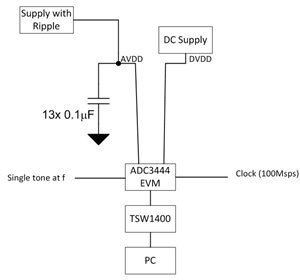Figure 7: Circuit configuration for a non-ideal AVDD supply

The system design tolerance will reveal the maximum acceptable spur to maintain the desired performance. Let’s consider here that the worst spur cannot exceed -95dBFS. This means that with a 16dB worst-case PSRR and using Equations 1 and 2 below, we can determine the maximum allowable power-supply ripple.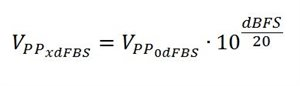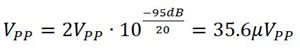(maximum allowable ripple amplitude after ADC PSRR attenuation)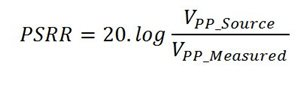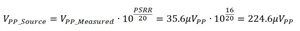This is the maximum ripple that can be present on the AVDD power-supply pin.

We can loosen this stringent requirement by:

• Reducing the guardband on the PSRR.
• Not operating the ADC at full dynamic range.
• Allowing spurs greater than -95dBFS in the FTT.

The DVDD supply PSRR would have the test configuration shown in Figure 8.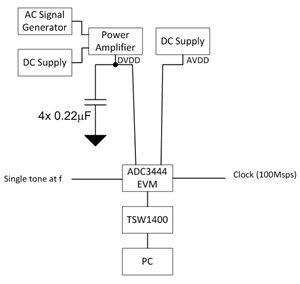Figure 8: Circuit configuration for a non-ideal AVDD supply

The DVDD is worst at 62dB. Maintaining a 10dB margin on this typical value, we can calculate that the worst disturber on the DVDD supply pin to ensure -95dBFS performance in the FFT is 14.17mVpp.

These calculations provide a guideline for the required performance of both power supplies for the ADC. In my next post, I’ll discuss developing an adequate power-supply solution for each supply and its impact on performance.